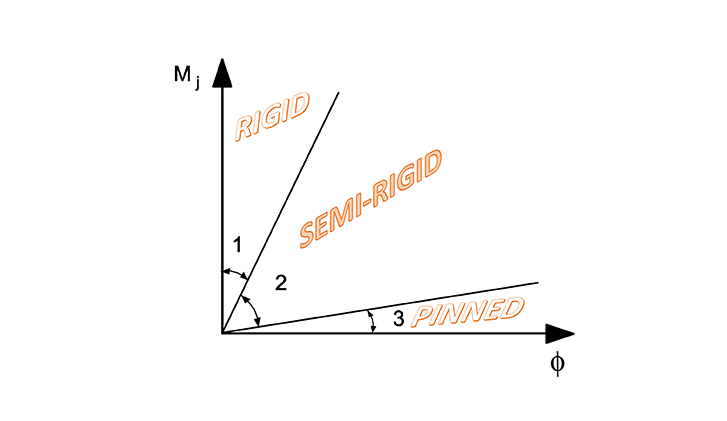HU# Semi-rigid joints in modeling of structures

## Introduction

The effects of the behaviour of the joints on the distribution of internal forces and moments within a structure, and on the overall deformations of the structure, should generally be taken into account, but where these effects are sufficiently small they may be neglected.

## Classification

In the case of elastic analysis, joints should be classified according to their rotational stiffness. The joints should have sufficient strength to transmit the forces and moments acting at the joints resulting from the analysis. A joint may be classified as rigid, pinned or semi-rigid, according to its rotational stiffness, by comparing its initial rotational stiffness Sj,ini with the classification boundaries given in EN1993-1-8 5.2.2.5. In the case of a semi-rigid joint, the rotational stiffness Sj corresponding to the bending moment MEd should generally be used in the analysis.

## Calculation of joint stiffness

Consteel Joint calculates the Sj,ini initial stiffness of the joint, the Sj,sec secant stiffness for the actual loading and determines the classes of stiffness and strength (last one for plastic analysis).

Sj,sec is equal to Sj,ini when MEd does not exceed 2/3*MjRd (EC3-1-8 5.1.2. (3) ), otherwise it is calculated.

## Automatic consideration of connection stiffness